Importance: High ✭✭✭
 Author(s): Hall, Marshall Paige, Lowell J.
 Subject: Group Theory
 Keywords: complete map finite group latin square
 Posted by: mdevos on: October 21st, 2007
 Solved by: Wilcox, Stewart

A complete map for a (multiplicative) groupis a bijection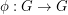so that the mapis also a bijection.

Conjecture   Ifis a finite group and the Sylow 2-subgroups ofare either trivial or non-cyclic, thenhas a complete map.

The motivation for this problem comes from the study of Latin Squares. Call a collection ofcells from a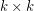matrix a transversal if there is exactly one cell from each row and one from each column, and say that a transversal is latin if no two of its cells contain the same element. The multiplication table of every (finite multiplicative) groupis a latin square, and we see thathas a latin transversal if and only if it has a complete map (the cells indexed by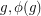form the transversal). If we do have such a latin transversal, then for every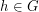, the map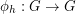given by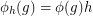is also a latin transversal, and the collection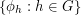gives uspairwise disjoint latin transversals in the multiplication table of. This is precisely equivalent to the existence of a latin square orthogonal to this multiplication table. Thus, the above conjecture may be restated as follows.

Conjecture  (Hall-Paige version 2)   Ifis a finite group and the Sylow 2-subgroups ofare either trivial or non-cyclic, then there is a latin square orthogonal to the multiplication table of.

Next, let us show that the assumption in the conjecture on Sylow 2-subgroups is necessary, so letbe a group with nontrivial cyclic 2-Sylow subgroup. Under these assumptions, it follows from a theorem of Burnside that there exists a normal subgroup(of odd order) so that. Let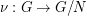be the canonical homomorphism and letbe the unique element in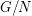of order 2. Now, suppose (for a contradiction) thathas a complete mapand consider the three families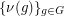,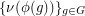,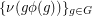, and their products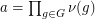,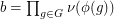, and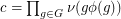. For everywe have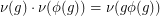, so taking the product of all of these equations we get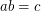(sinceis abelian, the order doesn't matter here). However, every element inoccurs exactlytimes in each of these families, and it follows from this that, giving us a contradiction.

Hall and Paige proved their conjecture for solvable groups, and for the alternating and symmetric groups. More recently, a number of authors have studied this problem, establishing a number of partial results. Dalla Volta and Gavioli [DG3] have shown that a minimal counterexample to the conjecture is either almost simple, or it has a unique central involution and its derived subgroup is is a quasi-simple group of index 2. The following list contains a number groups for which the problem is solved.

\item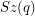,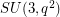,where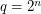and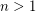[D] \item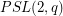,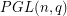,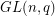withodd [DG1] [DG2] \item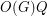whereis the largest normal subgroup of odd order and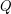is a Frobenius complement [D] \item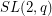[E2] [E3] [S] \item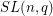,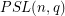,,witheven and[DG1] \item Matthieu groups and their automorphism groups [DG1]

The Hall-Paige conjecture is rather trivial for abelian groups, but Snevily has suggested an interesting conjecture concerning latin transversals in submatricies of the latin squares given by multiplication tables in abelian groups. There is a common generalization of these two conjectures highlighted below. I (M. DeVos) have no reason to suggest this conjecture other than its natural appearance, so it may well be false already for some small examples.

Conjecture   Letbe a group and letbe subsets ofwith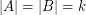. Then there exists an ordering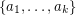ofand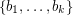ofso that the productsare distinct unless there is a subgroupso that the Sylow 2-subgroups ofare nontrivial and cyclic, and there exist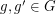so that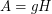and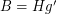.

## Bibliography

[W] Wilcox, Stewart, Reduction of the Hall-Paige conjecture to sporadic simple groups, J. Algebra 321 (2009), no. 5, 1407--1428. MathSciNet

[DG1] F. Dalla Volta, N. Gavioli, Complete mappings in some linear and projective groups, Arch. Math. (Basel) 61 (1993) 111--118. MathSciNet.

[DG2] F. Dalla Volta, N. Gavioli, On the admissibility of some linear and projective groups in odd characteristic, Geom. Dedicata 66 (1997) 245--254. MathSciNet.

[DG3] F. Dalla Volta, N. Gavioli, N. Minimal counterexamples to a conjecture of Hall and Paige. Arch. Math. (Basel) 77 (2001), no. 3, 209--214. MathSciNet.

[D] O. M. Di Vincenzo, On the existence of complete mappings of finite groups. Rend. Mat. 9, 7, 189--198 (1989). MathSciNet.

[E1] A.B. Evans, The existence of complete mappings of finite groups, Congr. Numer. 90 (1992) 65--75. MathSciNet.

[E2] A.B. Evans, The existence of complete mappings of,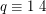, Finite Fields Appl. 7 (2001) 373--381. MathSciNet.

[E3] A. B. Evans, The existence of complete mappings of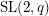,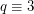modulo 4. Finite Fields Appl. 11 (2005), no. 1, 151--155. MathSciNet.

*[HP] M. Hall, L. J. Paige, Complete mappings of finite groups, Pacific J. Math. 5 (1955) 541--549. MathSciNet.

[S] D. Saeli, Saeli applicazioni complete e rapporto incrementale in doppi cappi. Riv. Mat. Univ. Parma 15, 4, 11--117 (1989). MathSciNet.

[WV] M. Vaughan-Lee, I. M. Wanless, Latin squares and the Hall-Paige conjecture. Bull. London Math. Soc. 35 (2003), no. 2, 191--195. MathSciNet.

* indicates original appearance(s) of problem.